Next: Newton method for solving Up: Brent method for solving Previous: Mathematical background   Contents

Implementation

Brent's method enable to find a root of an equation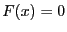as soon as the root is bracketed in an interval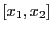such that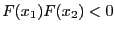. It is implemented as:

int Brent(REAL (* TheFunction)(REAL),INTERVAL &Input,
double AccuracyV,double Accuracy,int Max_Iter,double *Sol, double *Residu)
with:
• TheFunction: a procedure which enable to compute the value of the equation at a given point
• Input: the intervalin which we are looking for a root
• AccuracyF: a threshold on the minimal value of the width of the interval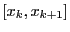with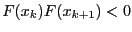considered during the procedure
• Accuracy: a threshold on the value of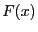which determine a root of the equation
• MaxIter: maximal number of iteration
• Sol: on success the value of the root
• Residu: the value of the equation at Sol
The procedure returns:
• 1: a solution has been found as F(Sol)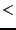Accuracy
• 2: a solution has been found as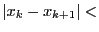AccuracyV
• -1: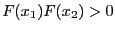• -3: the maximal number of iteration has been reached without finding a solution
The test program Test_Ridder2 present a program to solve the trigonometric equation presented as example 2 (see section 15.1.1).Next: Newton method for solving Up: Brent method for solving Previous: Mathematical background   Contents
Jean-Pierre Merlet 2012-12-20